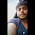# Series of Fibonacci and Prime numbers | 1, 2, 1, 3, 2, 5, 3, 7, 5, 11, 8, 13, 13, 17, … This series is a mixture of 2 series – all the odd terms in this series form a Fibonacci series and all the even terms are the prime numbers in ascending order.

Consider the below series:

1, 2, 1, 3, 2, 5, 3, 7, 5, 11, 8, 13, 13, 17, …

This series is a mixture of 2 series – all the odd terms in this series form a Fibonacci series and all the even terms are the prime numbers in ascending order.

Write a program to find the Nth term in this series.

The value N is a Positive integer that should be read from STDIN. The Nth term that is calculated by the program should be written to STDOUT. Other than the value of Nth term, no other characters/strings or message should be written to STDOUT.

For example, when N = 14, the 14th term in the series is 17. So only the value 17 should be printed to STDOUT.

#include<stdio.h>
#define MAX 1000
void fibonacci(int n)
{
int i, term1 = 0, term2 = 1, nextTerm;
for (i = 1; i<=n; i++)
{
nextTerm = term1 + term2;
term1 = term2;
term2 = nextTerm;
}
printf("%d", term1);
}

void prime(int n)
{
int i, j, flag, count =0;
for (i=2; i<=MAX; i++)
{
flag = 0;
for (j=2; j<i; j++)
{
if(i%j == 0)
{
flag = 1;
break;
}
}
if (flag == 0)
count++;
if(count == n)
{
printf("%d", i);
break;
}
}
}
int main( )
{
int n;
scanf("%d", &n);
if(n%2 == 1)
fibonacci (n/2 + 1);
else
prime(n/2);
return 0;
}

1.code is not work properly ...

1.https://ideone.com/ldihuz
I have executed it here....Check it out !!

2.Sorry , it's correct ,
My compiler have some issue ...